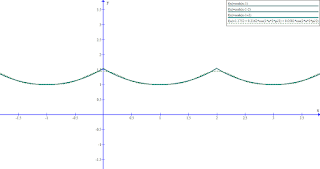## Friday, August 30, 2019

### The Fourier transform for real numbers (approximating complicated periodic functions with simple sinusoids)

In a previous post we saw how to approximate complex functions using simple polynomials by using the Taylor series approximation method. This consisted in assuming that your complex function was an actual polynomial and then using mathematical tricks to tease out the coefficients of the polynomial one by one. Polynomials are useful functions to approximate curves but are terrible at approximating periodic functions such as $sin(x)$ (repeat themselves periodically) because they themselves are not periodic. In this post we'll see how to approximating periodic functions by using a sum of simple sinusoids (sines or cosines). With polynomials, we had a summation of powers of $x$, each of which having a different power. Now we will instead be having a summation of sinusoids, each of which having a different whole number frequency. With polynomials we had to find the coefficients, which are constants multiplied by the power of $x$, and any curve could be arbitrarily approximated by finding the right coefficients. Now we will be finding amplitudes, which are constants multiplied by each sinusoid, and any periodic function can be arbitrarily approximated by finding the right amplitudes.

Instead of the Taylor series approximation we will now be using the Fourier series approximation, also known as the Fourier transform. In order to keep things simple we will only be looking at real functions rather than complex ones (functions with imaginary components). The Fourier series assumes that the periodic function we're deconstructing, $f(x)$, begins its period at 0 and ends it at $T$, after which $f(x)$ will continue repeating itself such that $f(T + k) = f(k)$. If we'd like our period to start at $x_0$ instead then we can easily just replace our periodic function with $f'(x)$ such that $f'(x) = f(x + x_0)$ and then subtract $x_0$ from $x$ again after getting the approximate function.

Let's say that we have a complex periodic function where the first period is between 0 and 2 and is defined as $y = \cosh(x-1)$. This is what it looks like: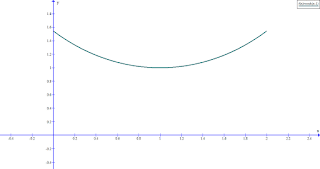What we mean by this being a periodic function is that if we looked beyond the first period we would see the function looking like this:We're assuming that our function consists of a summation of sinusoids with different frequencies and amplitudes such that each wavelength is equal to the period of the original function or a whole number division of that period (half, quarter, etc.). To make a sine or cosine have a period equal to $T$, we use $sin(x\frac{2\pi}{T})$ and $cos(x\frac{2\pi}{T})$. Multiplying $x$ by a whole number will change the frequency of the sinusoid such that the wavelength is equal to a whole number division of $T$. For example, $sin(2 x\frac{2\pi}{2})$ will make the sine function repeat itself twice when going from 0 to 2. This is what our sinusoid functions look like for both sine and cosine:By adding up these sines and cosines together whilst having specific amplitudes (that we need to find) we can get closer and closer to the original periodic function.

The Fourier transform takes advantage of an interesting quirk of sinusoids and the area under their graph. For two positive whole numbers $n$ and $m$, if you multiply $\sin(n x\frac{2\pi}{T})$ by $\sin(m x\frac{2\pi}{T})$ or $\cos(n x\frac{2\pi}{T})$ by $\cos(m x\frac{2\pi}{T})$, the area under the graph between 0 and $T$ will always be zero, with the only exception being when $n = m$. If $n = m$ then the area will be equal to $\frac{T}{2}$. In other words,

$$\int_{0}^{T} \sin\left(n x\frac{2\pi}{T}\right) \sin\left(m x\frac{2\pi}{T}\right) dx = \begin{cases} 0& \text{if } n \neq m\\ \frac{T}{2}& \text{otherwise} \end{cases}$$
and likewise for $\cos$ instead of $\sin$. Here's an example showing the area under the graph of two cosines with different frequencies being multiplied together: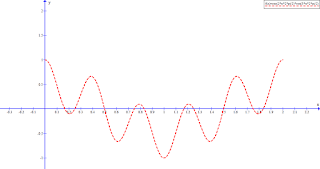Note how each hump above the x-axis has a corresponding anti-hump below the x-axis which cancel each other out, resulting in a total area of zero. Here's an example showing the area under the graph of two cosines with the same frequency being multiplied together: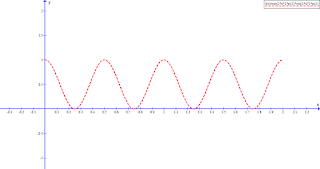Note how the area is all above the x-axis. If we had to measure this area, it would be equal to $\frac{T}{2}$ where $T = 2$. Let's prove this for all frequencies.

Proof that the area under the product of two sines with different frequencies is 0:
\begin{align} \int_{0}^{T} \sin\left(n x\frac{2\pi}{T}\right) \sin\left(m x\frac{2\pi}{T}\right) dx &= \int_{0}^{T} \frac{1}{2}\left(\cos\left(n x\frac{2\pi}{T} - m x\frac{2\pi}{T}\right) - \cos\left(n x\frac{2\pi}{T} + m x\frac{2\pi}{T}\right)\right) dx\\ &= \frac{1}{2}\left(\int_{0}^{T} \cos\left((n - m) \frac{2\pi}{T}x\right) dx - \int_{0}^{T} \cos\left((n + m) \frac{2\pi}{T}x\right) dx\right)\\ &= \frac{1}{2}\left(\frac{1}{(n - m) \frac{2\pi}{T}}\left[\sin\left((n - m) \frac{2\pi}{T}x\right)\right]_{0}^{T} - \frac{1}{(n + m) \frac{2\pi}{T}}\left[\sin\left((n + m) \frac{2\pi}{T}x\right)\right]_{0}^{T}\right)\\ &= \frac{1}{2}\left(\frac{1}{(n - m) \frac{2\pi}{T}}\left(\sin\left((n - m) \frac{2\pi}{T}T\right) - \sin\left((n - m) \frac{2\pi}{T}0\right)\right) - \frac{1}{(n + m) \frac{2\pi}{T}}\left(\sin\left((n + m) \frac{2\pi}{T}T\right) - \sin\left((n + m) \frac{2\pi}{T}0\right)\right)\right)\\ &= \frac{1}{2}\left(\frac{1}{(n - m) \frac{2\pi}{T}}\left(\sin\left((n - m) 2\pi\right) - \sin\left(0\right)\right) - \frac{1}{(n + m) \frac{2\pi}{T}}\left(\sin\left((n + m) 2\pi\right) - \sin\left(0\right)\right)\right)\\ &= \frac{1}{2}\left(\frac{1}{(n - m) \frac{2\pi}{T}}\left(\sin\left(2\pi\right) - \sin\left(0\right)\right) + \frac{1}{(n + m) \frac{2\pi}{T}}\left(\sin\left(2\pi\right) - \sin\left(0\right)\right)\right)\\ &= \frac{1}{2}\left(\frac{1}{(n - m) \frac{2\pi}{T}}\left(0 - 0\right) - \frac{1}{(n + m) \frac{2\pi}{T}}\left(0 - 0\right)\right)\\ &= 0\\ \end{align}

Proof that the area under the product of two sines with the same frequencies is half their period:
\begin{align} \int_{0}^{T} \sin\left(n x\frac{2\pi}{T}\right) \sin\left(n x\frac{2\pi}{T}\right) dx &= \int_{0}^{T} \sin^2\left(n x\frac{2\pi}{T}\right) dx\\ &= \int_{0}^{T} \frac{1}{2}\left(1 - \cos\left(2n x\frac{2\pi}{T}\right)\right) dx\\ &= \frac{1}{2}\left(\int_{0}^{T} 1 dx - \int_{0}^{T}\cos\left(2n\frac{2\pi}{T} x\right) dx\right)\\ &= \frac{1}{2}\left(\left[x \right]_{0}^{T} - \frac{1}{2n\frac{2\pi}{T}}\left[\sin\left(2n\frac{2\pi}{T} x\right)\right]_{0}^{T}\right)\\ &= \frac{1}{2}\left(\left(T - 0\right) - \frac{1}{2n\frac{2\pi}{T}}\left(\sin\left(2n\frac{2\pi}{T} T\right) - \sin\left(2n\frac{2\pi}{T} 0\right)\right)\right)\\ &= \frac{1}{2}\left(T - \frac{1}{2n\frac{2\pi}{T}}\left(\sin\left(2n2\pi\right) - \sin\left(0\right)\right)\right)\\ &= \frac{1}{2}\left(T - \frac{1}{2n\frac{2\pi}{T}}\left(\sin\left(2\pi\right) - \sin\left(0\right)\right)\right)\\ &= \frac{1}{2}\left(T - \frac{1}{2n\frac{2\pi}{T}}\left(0 - 0\right)\right)\\ &= \frac{T}{2}\\ \end{align}

Proof that the area under the product of two cosines with different frequencies is 0:
\begin{align} \int_{0}^{T} \cos\left(n x\frac{2\pi}{T}\right) \cos\left(m x\frac{2\pi}{T}\right) dx &= \int_{0}^{T} \frac{1}{2}\left(\cos\left(n x\frac{2\pi}{T} - m x\frac{2\pi}{T}\right) + \cos\left(n x\frac{2\pi}{T} + m x\frac{2\pi}{T}\right)\right) dx\\ &= \frac{1}{2}\left(\int_{0}^{T} \cos\left((n - m) \frac{2\pi}{T}x\right) dx + \int_{0}^{T} \cos\left((n + m) \frac{2\pi}{T}x\right) dx\right)\\ &= \frac{1}{2}\left(\frac{1}{(n - m) \frac{2\pi}{T}}\left[\sin\left((n - m) \frac{2\pi}{T}x\right)\right]_{0}^{T} + \frac{1}{(n + m) \frac{2\pi}{T}}\left[\sin\left((n + m) \frac{2\pi}{T}x\right)\right]_{0}^{T}\right)\\ &= \frac{1}{2}\left(\frac{1}{(n - m) \frac{2\pi}{T}}\left(\sin\left((n - m) \frac{2\pi}{T}T\right) - \sin\left((n - m) \frac{2\pi}{T}0\right)\right) + \frac{1}{(n + m) \frac{2\pi}{T}}\left(\sin\left((n + m) \frac{2\pi}{T}T\right) - \sin\left((n + m) \frac{2\pi}{T}0\right)\right)\right)\\ &= \frac{1}{2}\left(\frac{1}{(n - m) \frac{2\pi}{T}}\left(\sin\left((n - m) 2\pi\right) - \sin\left(0\right)\right) + \frac{1}{(n + m) \frac{2\pi}{T}}\left(\sin\left((n + m) 2\pi\right) - \sin\left(0\right)\right)\right)\\ &= \frac{1}{2}\left(\frac{1}{(n - m) \frac{2\pi}{T}}\left(\sin\left(2\pi\right) - \sin\left(0\right)\right) + \frac{1}{(n + m) \frac{2\pi}{T}}\left(\sin\left(2\pi\right) - \sin\left(0\right)\right)\right)\\ &= \frac{1}{2}\left(\frac{1}{(n - m) \frac{2\pi}{T}}\left(0 - 0\right) + \frac{1}{(n + m) \frac{2\pi}{T}}\left(0 - 0\right)\right)\\ &= 0\\ \end{align}

Proof that the area under the product of two cosines with the same frequencies is half their period:
\begin{align} \int_{0}^{T} \cos\left(n x\frac{2\pi}{T}\right) \cos\left(n x\frac{2\pi}{T}\right) dx &= \int_{0}^{T} \cos^2\left(n x\frac{2\pi}{T}\right) dx\\ &= \int_{0}^{T} \frac{1}{2}\left(1 + \cos\left(2n x\frac{2\pi}{T}\right)\right) dx\\ &= \frac{1}{2}\left(\int_{0}^{T} 1 dx + \int_{0}^{T}\cos\left(2n\frac{2\pi}{T} x\right) dx\right)\\ &= \frac{1}{2}\left(\left[x \right]_{0}^{T} + \frac{1}{2n\frac{2\pi}{T}}\left[\sin\left(2n\frac{2\pi}{T} x\right)\right]_{0}^{T}\right)\\ &= \frac{1}{2}\left(\left(T - 0\right) + \frac{1}{2n\frac{2\pi}{T}}\left(\sin\left(2n\frac{2\pi}{T} T\right) - \sin\left(2n\frac{2\pi}{T} 0\right)\right)\right)\\ &= \frac{1}{2}\left(T + \frac{1}{2n\frac{2\pi}{T}}\left(\sin\left(2n2\pi\right) - \sin\left(0\right)\right)\right)\\ &= \frac{1}{2}\left(T + \frac{1}{2n\frac{2\pi}{T}}\left(\sin\left(2\pi\right) - \sin\left(0\right)\right)\right)\\ &= \frac{1}{2}\left(T + \frac{1}{2n\frac{2\pi}{T}}\left(0 - 0\right)\right)\\ &= \frac{T}{2}\\ \end{align}

Also interestingly, proof that the area under the product of a sine and cosine, regardless of frequency, is 0:
\begin{align} \int_{0}^{T} \sin\left(n x\frac{2\pi}{T}\right) \cos\left(m x\frac{2\pi}{T}\right) dx &= \int_{0}^{T} \frac{1}{2}\left(\sin\left(n x\frac{2\pi}{T} + m x\frac{2\pi}{T}\right) + \sin\left(n x\frac{2\pi}{T} - m x\frac{2\pi}{T}\right)\right) dx\\ &= \frac{1}{2}\left(\int_{0}^{T} \sin\left((n + m) \frac{2\pi}{T}x\right) dx + \int_{0}^{T} \sin\left((n - m) \frac{2\pi}{T}x\right) dx\right)\\ &= \frac{1}{2}\left(\frac{1}{(n + m) \frac{2\pi}{T}}\left[\sin\left((n + m) \frac{2\pi}{T}x\right)\right]_{0}^{T} + \frac{1}{(n - m) \frac{2\pi}{T}}\left[\sin\left((n - m) \frac{2\pi}{T}x\right)\right]_{0}^{T}\right)\\ &= \frac{1}{2}\left(\frac{1}{(n + m) \frac{2\pi}{T}}\left(\sin\left((n + m) \frac{2\pi}{T}T\right) - \sin\left((n + m) \frac{2\pi}{T}0\right)\right) + \frac{1}{(n - m) \frac{2\pi}{T}}\left(\sin\left((n - m) \frac{2\pi}{T}T\right) - \sin\left((n - m) \frac{2\pi}{T}0\right)\right)\right)\\ &= \frac{1}{2}\left(\frac{1}{(n + m) \frac{2\pi}{T}}\left(\sin\left((n + m) 2\pi\right) - \sin\left(0\right)\right) + \frac{1}{(n - m) \frac{2\pi}{T}}\left(\sin\left((n - m) 2\pi\right) - \sin\left(0\right)\right)\right)\\ &= \frac{1}{2}\left(\frac{1}{(n + m) \frac{2\pi}{T}}\left(\sin\left(2\pi\right) - \sin\left(0\right)\right) + \frac{1}{(n - m) \frac{2\pi}{T}}\left(\sin\left(2\pi\right) - \sin\left(0\right)\right)\right)\\ &= \frac{1}{2}\left(\frac{1}{(n + m) \frac{2\pi}{T}}\left(0 - 0\right) + \frac{1}{(n - m) \frac{2\pi}{T}}\left(0 - 0\right)\right)\\ &= 0\\ \end{align}

Great! Now we can get back to the Fourier transform. Suppose that we have the following sum of sines and cosines:
\begin{align} f(x) = &a_1 \cos\left(1 x\frac{2\pi}{T}\right) + b_1 \sin\left(1 x\frac{2\pi}{T}\right) + \\ &a_2 \cos\left(2 x\frac{2\pi}{T}\right) + b_2 \sin\left(2 x\frac{2\pi}{T}\right) + \\ &\dots \end{align}

How can we tease out the individual amplitudes $a_i$ and $b_i$? Thanks to the identities we proved above, we can now get any amplitude we want by multiplying the function by a sine or cosine, finding the area under the graph, and dividing the area by $\frac{T}{2}$. Here's how it works:
\begin{align} \frac{2}{T}\int_0^T \cos\left(n x\frac{2\pi}{T}\right) f(x) dx &= \frac{1}{2T}\int_0^T \cos\left(n x\frac{2\pi}{T}\right) \begin{pmatrix} &a_1 cos\left(1 x\frac{2\pi}{T}\right) & + b_1 sin\left(1 x\frac{2\pi}{T}\right) + \\ &a_2 cos\left(2 x\frac{2\pi}{T}\right) & + b_2 sin\left(2 x\frac{2\pi}{T}\right) + \\ &\dots& \end{pmatrix} dx\\ &= \frac{2}{T} \begin{pmatrix} &a_1 \int_0^T\cos\left(n x\frac{2\pi}{T}\right) cos\left(1 x\frac{2\pi}{T}\right) dx & + b_1 \int_0^T\cos\left(n x\frac{2\pi}{T}\right) sin\left(1 x\frac{2\pi}{T}\right) dx + \\ &\dots&\\ &a_n \int_0^T\cos\left(n x\frac{2\pi}{T}\right) cos\left(n x\frac{2\pi}{T}\right) dx & + b_n \int_0^T\cos\left(n x\frac{2\pi}{T}\right) sin\left(n x\frac{2\pi}{T}\right) dx + \\ &\dots& \end{pmatrix}\\ &= \frac{2}{T} \begin{pmatrix} &a_1 0 & + b_1 0 + \\ &\dots&\\ &a_n \frac{T}{2} & + b_n 0 + \\ &\dots& \end{pmatrix}\\ &= \frac{2}{T} a_n \frac{T}{2}\\ &= a_n \end{align}

And obviously multiplying by $\sin\left(n x\frac{2\pi}{T}\right)$ would yield the amplitude of a sine. All that's left is by how much to vertically lift the graph, that is, the constant term to add to the sinusoids. The sinusoids should oscillate around the average value of the original function in one period, which is found as follows:

$y_0 = \frac{1}{T} \int_0^T f(x) dx$

Getting back to $f(x) = \cosh(x-1)$, here are the amplitudes:

$y_0 = \frac{1}{2} \int_0^2 f(x) dx = 1.1752$
$a_1 = \frac{2}{2} \int_0^2 \cos\left(1 x\frac{2\pi}{2}\right) f(x) dx = 0.2162$
$b_1 = \frac{2}{2} \int_0^2 \sin\left(1 x\frac{2\pi}{2}\right) f(x) dx = 0.0$
$a_2 = \frac{2}{2} \int_0^2 \cos\left(2 x\frac{2\pi}{2}\right) f(x) dx = 0.0581$
$b_2 = \frac{2}{2} \int_0^2 \sin\left(2 x\frac{2\pi}{2}\right) f(x) dx = 0.0$

And here are the graphs of the approximated function getting better with every new term (where the approximated function is denoted as $\hat{f}$):

$\hat{f}(x) = 1.1752$$\hat{f}(x) = 1.1752 + 0.2162 \cos\left(1 x\frac{2\pi}{2}\right)$$\hat{f}(x) = 1.1752 + 0.2162\cos\left(1 x\frac{2\pi}{2}\right) + 0.0581\cos\left(2 x\frac{2\pi}{2}\right)$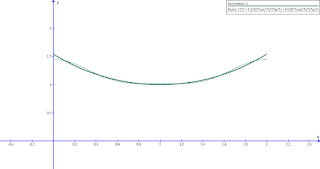And of course here is the function beyond the first period: